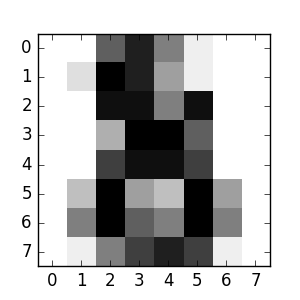# 使用scikit-learn进行机器学习的简介¶

## 机器学习：问题设置¶

• 监督学习 ，其中数据带有我们要预测的附加属性，（ 点击这里 去scikit-learn监督学习的页面）。这个问题可以是：

• 分类 ：样本属于两个或多个类，我们想要从已标记的数据中学习如何预测未标记的数据类别。分类问题的一个例子是手写体数字识别，其中目标是将每个输入向量指派到一个有限数量的离散类。另一种方式去理解分类，其实分类问题是一种离散（相对于连续）形式的监督学习，每一个都有一个有限数量的类别，对每个提供的样本，我们要尝试用正确的类别来标注他们。

• 回归 ：如果期望的输出由一个或多个连续变量组成，那么这个任务被称为*回归*。回归问题的一个例子是，鲑鱼长度的预测——一个基于其年龄和体重的函数。

• 无监督学习 ，训练数据包含一组没有相应目标值的输入向量x。这类问题的目标可能是在数据中发现相似的样本组，也被称为 聚类 ；或是在输入空间中确定数据的分布，也被称为 密度估计 ；或是把数据从高维空间投影到两个或三个维度的空间来达到*可视化*的目的（ 点击这里 去scikit-learn无监督学习的页面）。

## 加载样例数据集¶

scikit-learn 有一些标准数据集，例如用于分类的 irisdigits 数据集，用于回归的 boston house prices dataset 数据集。

```\$ python
>>> from sklearn import datasets
```

```>>> print(digits.data)
[[  0.   0.   5. ...,   0.   0.   0.]
[  0.   0.   0. ...,  10.   0.   0.]
[  0.   0.   0. ...,  16.   9.   0.]
...,
[  0.   0.   1. ...,   6.   0.   0.]
[  0.   0.   2. ...,  12.   0.   0.]
[  0.   0.  10. ...,  12.   1.   0.]]
```

`digits.target` 则是digits数据集的真实值，即我们尝试学习的每个数字图像对应的数字：

```>>> digits.target
array([0, 1, 2, ..., 8, 9, 8])
```

```>>> digits.images
array([[  0.,   0.,   5.,  13.,   9.,   1.,   0.,   0.],
[  0.,   0.,  13.,  15.,  10.,  15.,   5.,   0.],
[  0.,   3.,  15.,   2.,   0.,  11.,   8.,   0.],
[  0.,   4.,  12.,   0.,   0.,   8.,   8.,   0.],
[  0.,   5.,   8.,   0.,   0.,   9.,   8.,   0.],
[  0.,   4.,  11.,   0.,   1.,  12.,   7.,   0.],
[  0.,   2.,  14.,   5.,  10.,  12.,   0.,   0.],
[  0.,   0.,   6.,  13.,  10.,   0.,   0.,   0.]])
```

## 学习和预测¶

```>>> from sklearn import svm
>>> clf = svm.SVC(gamma=0.001, C=100.)
```

```>>> clf.fit(digits.data[:-1], digits.target[:-1])
SVC(C=100.0, cache_size=200, class_weight=None, coef0=0.0,
decision_function_shape=None, degree=3, gamma=0.001, kernel='rbf',
max_iter=-1, probability=False, random_state=None, shrinking=True,
tol=0.001, verbose=False)
```

```>>> clf.predict(digits.data[-1:])
array()
```## 模型持久化¶

```>>> from sklearn import svm
>>> from sklearn import datasets
>>> clf = svm.SVC()
>>> X, y = iris.data, iris.target
>>> clf.fit(X, y)
SVC(C=1.0, cache_size=200, class_weight=None, coef0=0.0,
decision_function_shape=None, degree=3, gamma='auto', kernel='rbf',
max_iter=-1, probability=False, random_state=None, shrinking=True,
tol=0.001, verbose=False)

>>> import pickle
>>> s = pickle.dumps(clf)
>>> clf2.predict(X[0:1])
array()
>>> y
0
```

```>>> from sklearn.externals import joblib
>>> joblib.dump(clf, 'filename.pkl')
```

```>>> clf = joblib.load('filename.pkl')
```

joblib.dump返回一个文件名列表。每一个被包含在 `clf` 对象中的numpy数组都被序列化为文件系统上的一个单独文件。用joblib.load重新加载模型时，所有文件都需要在同一个文件夹中。

## 惯例约定¶

scikit-learn估计量遵循一定的规则，使它们的行为更具有预测性。

### 类型转换¶

```>>> import numpy as np
>>> from sklearn import random_projection

>>> rng = np.random.RandomState(0)
>>> X = rng.rand(10, 2000)
>>> X = np.array(X, dtype='float32')
>>> X.dtype
dtype('float32')

>>> transformer = random_projection.GaussianRandomProjection()
>>> X_new = transformer.fit_transform(X)
>>> X_new.dtype
dtype('float64')
```

```>>> from sklearn import datasets
>>> from sklearn.svm import SVC
>>> clf = SVC()
>>> clf.fit(iris.data, iris.target)
SVC(C=1.0, cache_size=200, class_weight=None, coef0=0.0,
decision_function_shape=None, degree=3, gamma='auto', kernel='rbf',
max_iter=-1, probability=False, random_state=None, shrinking=True,
tol=0.001, verbose=False)

>>> list(clf.predict(iris.data[:3]))
[0, 0, 0]

>>> clf.fit(iris.data, iris.target_names[iris.target])
SVC(C=1.0, cache_size=200, class_weight=None, coef0=0.0,
decision_function_shape=None, degree=3, gamma='auto', kernel='rbf',
max_iter=-1, probability=False, random_state=None, shrinking=True,
tol=0.001, verbose=False)

>>> list(clf.predict(iris.data[:3]))
['setosa', 'setosa', 'setosa']
```

### 重新拟合和更新参数¶

```>>> import numpy as np
>>> from sklearn.svm import SVC

>>> rng = np.random.RandomState(0)
>>> X = rng.rand(100, 10)
>>> y = rng.binomial(1, 0.5, 100)
>>> X_test = rng.rand(5, 10)

>>> clf = SVC()
>>> clf.set_params(kernel='linear').fit(X, y)
SVC(C=1.0, cache_size=200, class_weight=None, coef0=0.0,
decision_function_shape=None, degree=3, gamma='auto', kernel='linear',
max_iter=-1, probability=False, random_state=None, shrinking=True,
tol=0.001, verbose=False)
>>> clf.predict(X_test)
array([1, 0, 1, 1, 0])

>>> clf.set_params(kernel='rbf').fit(X, y)
SVC(C=1.0, cache_size=200, class_weight=None, coef0=0.0,
decision_function_shape=None, degree=3, gamma='auto', kernel='rbf',
max_iter=-1, probability=False, random_state=None, shrinking=True,
tol=0.001, verbose=False)
>>> clf.predict(X_test)
array([0, 0, 0, 1, 0])
```